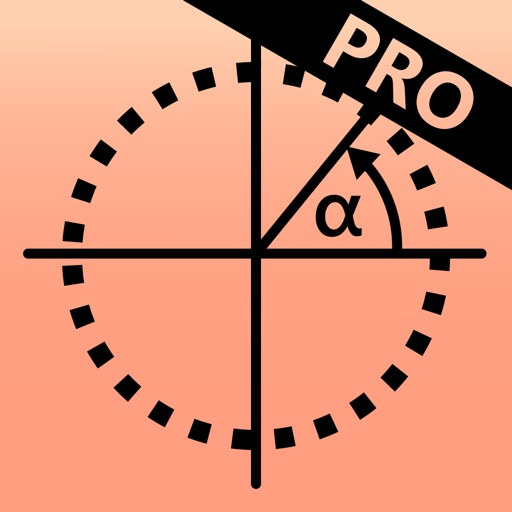## The unit circle is visualized and explained with calculation steps, diagrams and animations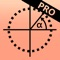# Unit Circle Calculator PRO

by Flooki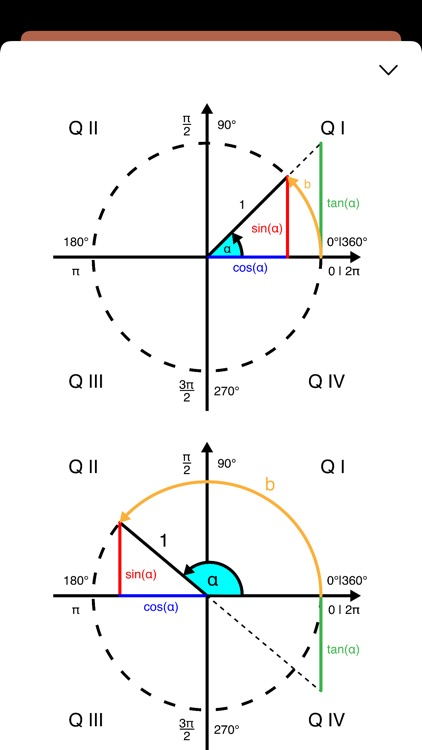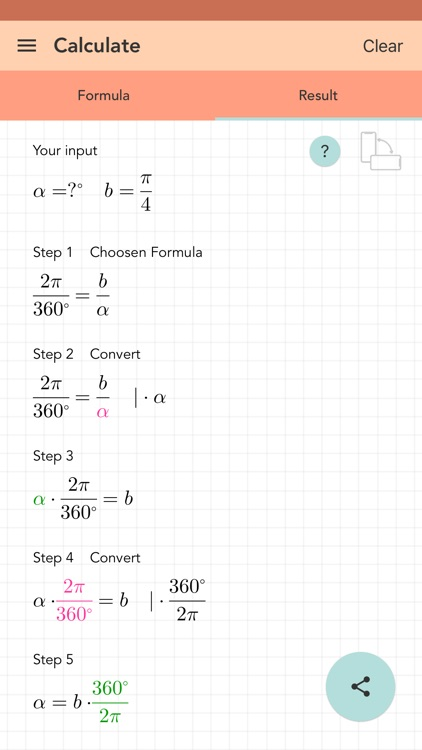The unit circle is visualized and explained with calculation steps, diagrams and animations. Enter the radian or the angle α to calculate the results.### App Details

Version
1.11
Rating
(12)
Size
29Mb
Genre
Education Utilities
Last updated
November 14, 2022
Release date
February 11, 2022

### App Screenshots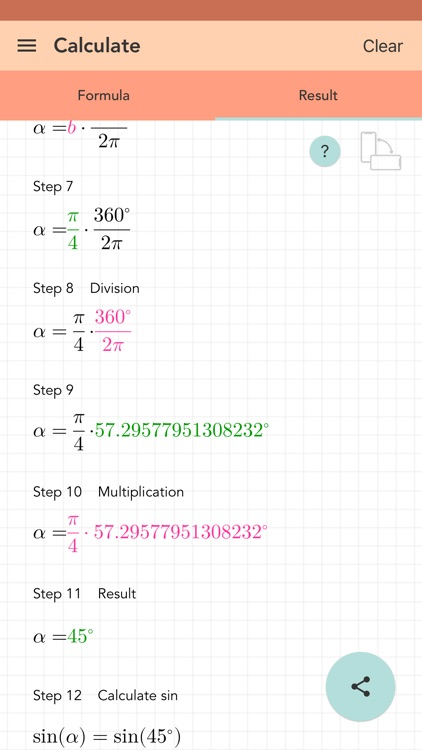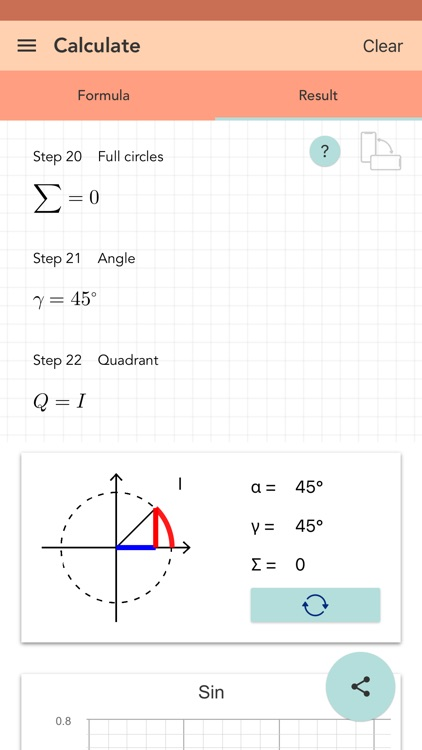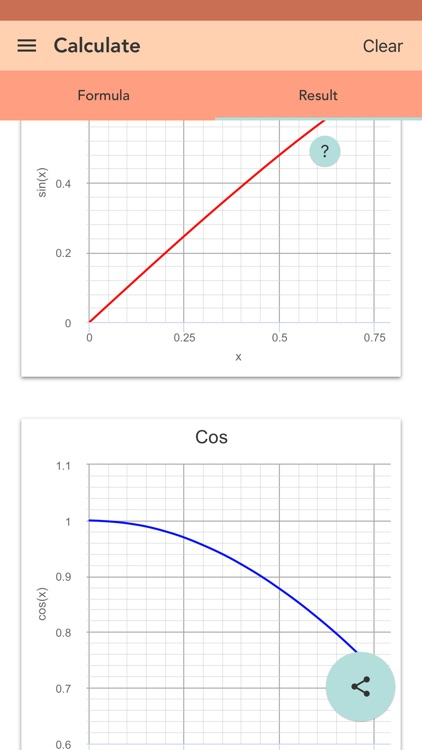### App Store Description

The unit circle is visualized and explained with calculation steps, diagrams and animations. Enter the radian or the angle α to calculate the results.

Negative values, decimals, fractions and π are supported. The solution is displayed step by step. All calculations are saved in the history. The solution can be shared.

[ Contents]
- all important quantities for the unit circle
- results are also calculated as π-value
- animation to show the angle α
- diagrams for sine and cosine
- history function to save the input
- detailed solution
- negative values, decimal numbers, fractions and π are supported